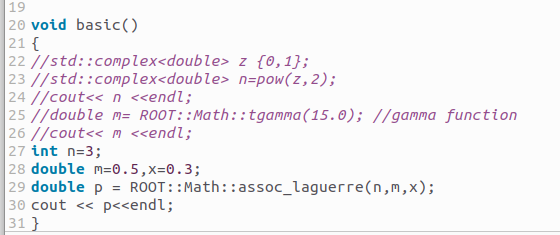# When I operate this simple file,there is such a problem.I don't know how to solve it. Could someone help me?can you copy/paste it ?

``````void basic()
{
//std::complex<double> z {0,1};
//std::complex<double> n=pow(z,2);
//cout<< n <<endl;
//double m= ROOT::Math::tgamma(15.0); //gamma function
//cout<< m <<endl;
int n=3;
double m=0.5,x=0.3;
double p = ROOT::Math::assoc_laguerre(n,m,x);
cout << p<<endl;
}
``````

I guess @moneta can help.

Thank you for your advice! Just now , I changed “p=ROOT::Math::assoc_laguerre(n,m,x)” to “p=assoc_laguerre(n,m,x)”.I got the value.

Make sure you understand that the “`ROOT::Math::assoc_laguerre(unsigned n, double m, double x)`” is not exactly the same as the “`assoc_laguerre(unsigned n, unsigned m, double x)`” mathematical special function provided by the C++ library (note: your “`double m = 0.5`” will be taken as “`unsigned m = 0`”).

BTW. For the missing GSL library, you need to install an appropriate package, e.g., on Ubuntu / Debian try: `sudo apt install libgsl-dev`

1 Like

Thanks for your advice. After I did this, my problem was solved. Maybe I am missing GSL library.

This topic was automatically closed 14 days after the last reply. New replies are no longer allowed.